# GEOGRAPHICAL DATABASE Tminus 2 Geographical Databases Post GIS

• Slides: 12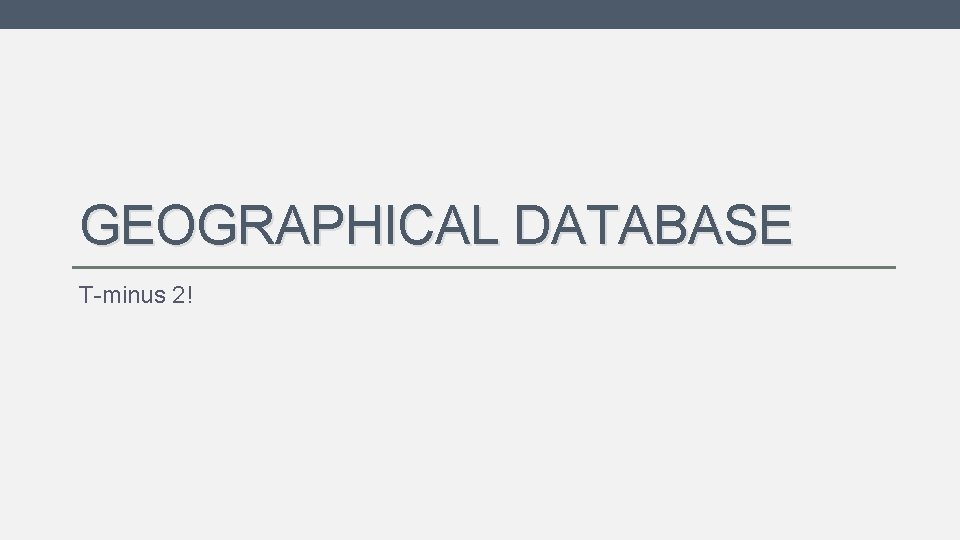GEOGRAPHICAL DATABASE T-minus 2!Geographical Databases • Post. GIS: • Postgre. SQL (object-relational database system) with support for geographic objects and GIS features. • WKT format • Well Known Text, special syntax used in Post. GIS to describe geographical objects (Points, lines, polygons, …) • Geo-operators and functions on Post. GIS • WKT to Web objectsPost. GIS - WKT • Post. GIS adds the geometry type to an database catalog. • Uses Well-Known Binary format (WKB) to store Well-Known text (WKT). WKT syntax examples: POINT(0 0) LINESTRING(0 0, -1 1, 2 2) Linestrings are equivalent to Google Maps’ polylines POLYGON((0 0, 4 4, 0 0), (1 1, 2 2, 1 1)) MULTIPOINT((0 0), (1 2), (-1 1), (-2 4)) MULTILINESTRING((0 0, 1 1, 1 2), (2 3, 3 2, 5 4)) MULTIPOLYGON(((0 0, 4 4, 0 0), (1 1, 2 2, 1 1)), ((-1 -1, -1 -2, -2 -1, -1 -1))) GEOMETRYCOLLECTION(POINT(2 3), LINESTRING(2 3, 3 4))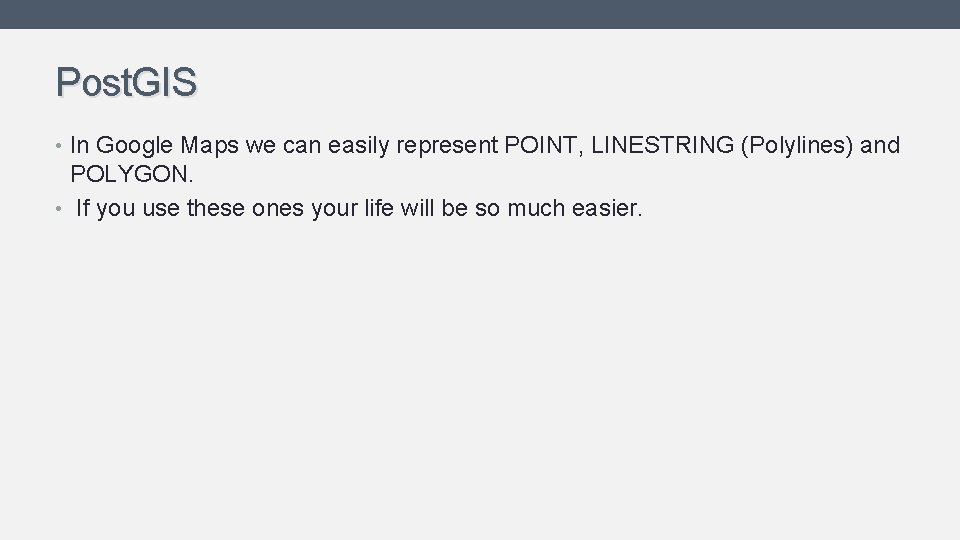Post. GIS • In Google Maps we can easily represent POINT, LINESTRING (Polylines) and POLYGON. • If you use these ones your life will be so much easier.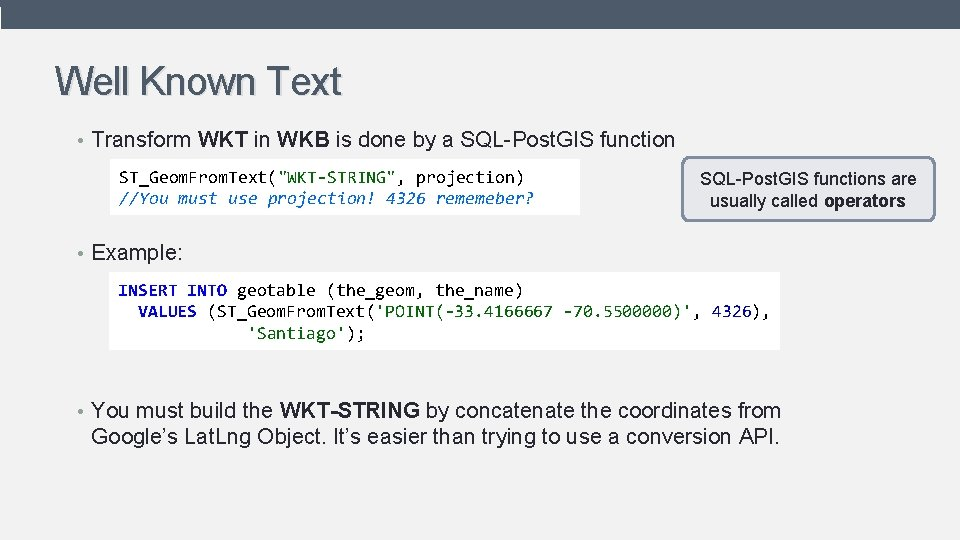Well Known Text • Transform WKT in WKB is done by a SQL-Post. GIS function ST_Geom. From. Text("WKT-STRING", projection) //You must use projection! 4326 rememeber? SQL-Post. GIS functions are usually called operators • Example: INSERT INTO geotable (the_geom, the_name) VALUES (ST_Geom. From. Text('POINT(-33. 4166667 -70. 5500000)', 4326), 'Santiago'); • You must build the WKT-STRING by concatenate the coordinates from Google’s Lat. Lng Object. It’s easier than trying to use a conversion API.Post. GIS Operators Operator Description ST_Distance(geometry, geometry) Return the cartesian distance between two geometries in projected units. ST_Intersects(geometry, geometry) Returns 1 (TRUE) if the Geometries spatially intersect. ST_Within(geometry A, geometry B) ) Returns 1 (TRUE) if Geometry A is spatially within Geometry B. A has to be completely inside B. // A is a polygon ST_Contains(geometry A, geometry B) // A is a polygon Returns 1 (TRUE) if Geometry A spatially contains Geometry B. ST_Centroid(geometry) Returns the centroid of the geometry as a point. ST_Length(geometry) The length of this Curve in its spatial reference. More about operators in: http: //postgis. refractions. net/docume ntation/manual-1. 3/ch 06. htmlPost. GIS Operators • Example of Operators: SELECT geotable. the_name FROM geotable WHERE ST_Distance( ST_Geom. From. Text('POINT(-33. 4166667 -70. 5500000)', 4326), geotable. the_geom) < 1000; Both geometries must be using the same projection • Distance is relative to the coordinate system, so in order to get meters: ST_Distance( ST_Transform(ST_Geom. From. Text('geom 1', 4326), 26986), ST_Transform(ST_Geom. From. Text('geom 2', 4326), 26986) ); 26986 is a coordinate system that uses meters. Not compatible with Google Maps.Post. GIS results to Google Maps • Lets assume that the resulting WKT is already loaded into a Javascript variable on the browser. (In the next section this will be done by creating a web-service). var mywkt = 'POINT(-33. 4166667 -70. 5500000)'; // easy to parse, but not so much if it’s a linestring or a polygon. • Google Maps does not read WKT format, so in order to do that we are going to use Open. Layers API to parse, and then we are going to convert manually from Open. Layers object to Google Maps objects.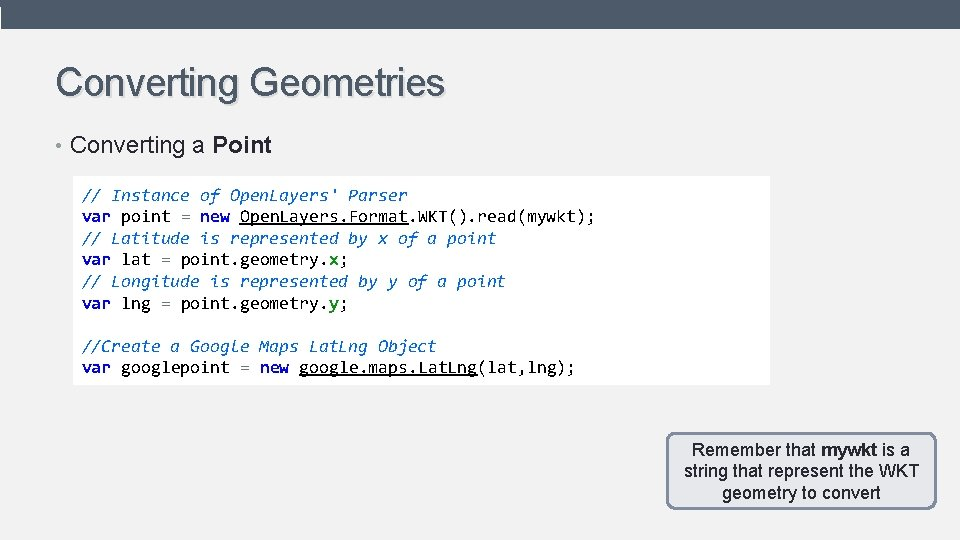Converting Geometries • Converting a Point // Instance of Open. Layers' Parser var point = new Open. Layers. Format. WKT(). read(mywkt); // Latitude is represented by x of a point var lat = point. geometry. x; // Longitude is represented by y of a point var lng = point. geometry. y; //Create a Google Maps Lat. Lng Object var googlepoint = new google. maps. Lat. Lng(lat, lng); Remember that mywkt is a string that represent the WKT geometry to convertConverting Geometries • Converting a Line. String //Instance of Open. Layers' Parser var result = new Open. Layers. Format. WKT(). read(mywkt); var latlng. Array = []; // Each point of linestring is represented in geometry. components array for(var i in results. geometry. components){ var lat = results. geometry. components[i]. x; var lng = results. geometry. components[i]. y; latlng. Array[i] = new google. maps. Lat. Lng(lat, lng); } //Create a Polyline Object var polyline = new google. maps. Polyline({ path: latlng. Array, //Array of Lat. Lng map: map, //Map Object });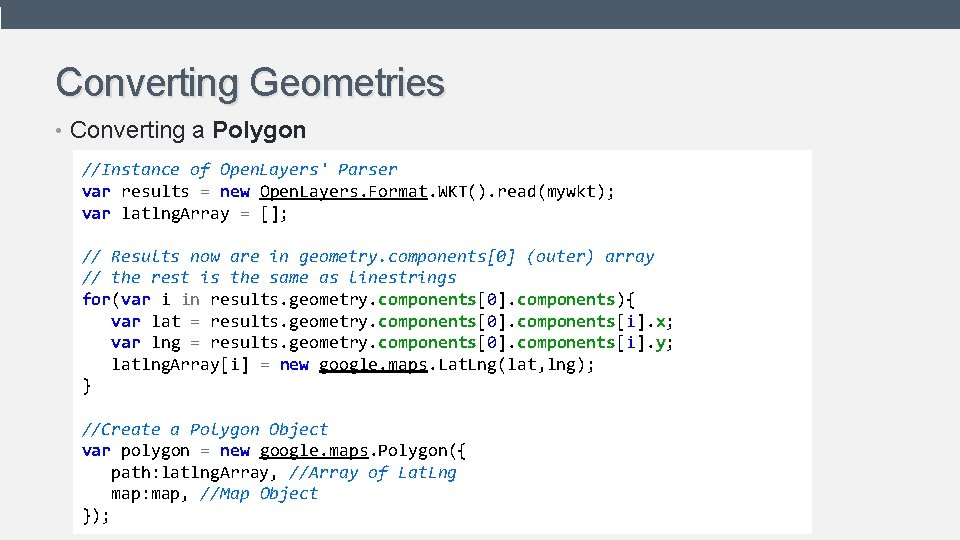Converting Geometries • Converting a Polygon //Instance of Open. Layers' Parser var results = new Open. Layers. Format. WKT(). read(mywkt); var latlng. Array = []; // Results now are in geometry. components (outer) array // the rest is the same as linestrings for(var i in results. geometry. components. components){ var lat = results. geometry. components. components[i]. x; var lng = results. geometry. components. components[i]. y; latlng. Array[i] = new google. maps. Lat. Lng(lat, lng); } //Create a Polygon Object var polygon = new google. maps. Polygon({ path: latlng. Array, //Array of Lat. Lng map: map, //Map Object });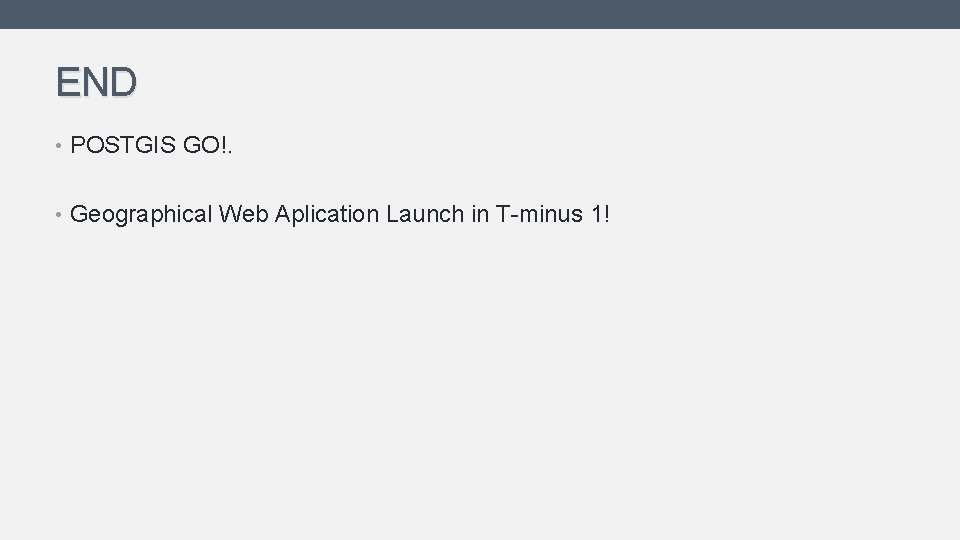END • POSTGIS GO!. • Geographical Web Aplication Launch in T-minus 1!